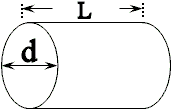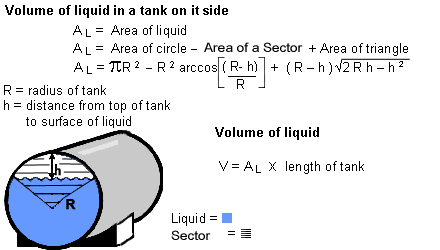# Horizontal Cylindrical Tank Volume Calculator

The outcomes pertain to:

• a perfectly cylindrical horizontal tank,
• with completely flat front and rear ends,
• which is not tilted in any way,
• which can be filled to the hilt.
In practice, many tanks are more oval than cylindrical, have rounded or coned ends, are tilted towards the outlet, en can not be filled to the hilt, because of manholes, agitators or other outlets at the top of the tank.
Therefore the outcomes of the calculations on this page can only be used for indicative purposes. It might help you to estimate the content of a tank.

Do not use the outcomes to ascertain the commercial value of the contents of a tank. For trustworthy measuring of the liquid volume inside a particular tank, the tank must be calibrated by professionals of the local official calibration authorities.

### Total volume calculation of a cylindrical tank

The calculation tool below makes it easy to determine the maximum volume of a cylinder-shaped tank. The "Create Dipstick Chart"-button can be used to derive a simple chart, for a quick reference to the volume of a partially filled round tank, when the fluid depth, and the tank dimensions are known.

## Tank Volume Calculator

All dimensions are in centimeters, volume is in litres (rounded number).
Both diameter and length must be entered & calculated first in order to create a corresponding Dipstick Chart.

 Determine Maximum VolumeTank Diameter d (in cm.): Tank Length L (in cm.): Volume (in ltr.):
(use a dot ( . ) as a decimal point to compute with non-integer values)

(opens a new window)

### Some Theory### Using the theory

Use this calculator for computing the volume of partially-filled horizontal cylinder-shaped tanks. With horizontal cylinders, volume changes are not linear and in fact are rather complex as the theory above shows. Fortunately you have this tool to do the work for you.
This calculator can provide a single detailed value of the volume of a partially filled horizontal round tank. Just insert the tank diameter, the tank length, and the reading on the dip stick, ruler, or measuring tape.

## Horizontal Tank Level Calculator

All dimensions are in meters or feet, volume is in liters or gallons.
The depth of the liquid in the tank, as read on the dipstick, should be filled in at the Liquid Level box.>>>>> >>>>> >>>>> or
(use a dot ( . ) as a decimal point to compute with non-integer values)